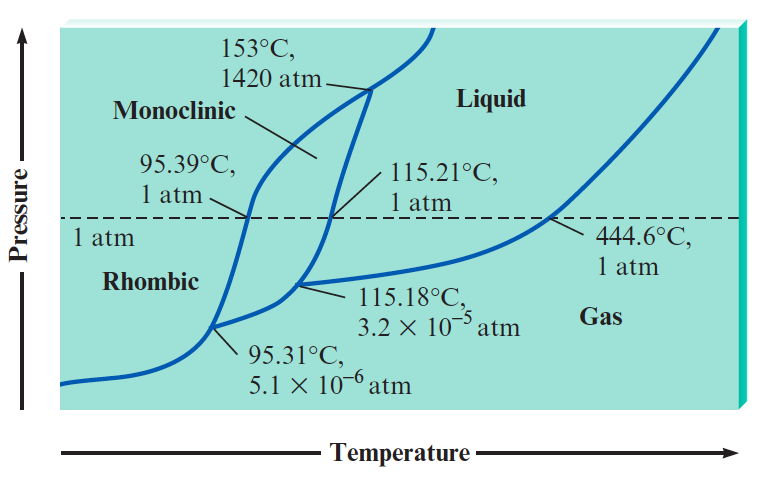# Problem: Sulfur exhibits two solid phases, rhombic and monoclinic. Use the accompanying phase diagram for sulfur to answer the following questions. (The phase diagram is not to scale.)What are the normal melting point and the normal boiling point of sulfur?

95% (13 ratings)
###### Problem Details

Sulfur exhibits two solid phases, rhombic and monoclinic. Use the accompanying phase diagram for sulfur to answer the following questions. (The phase diagram is not to scale.)What are the normal melting point and the normal boiling point of sulfur?

Frequently Asked Questions

What scientific concept do you need to know in order to solve this problem?

Our tutors have indicated that to solve this problem you will need to apply the Phase Diagram concept. You can view video lessons to learn Phase Diagram. Or if you need more Phase Diagram practice, you can also practice Phase Diagram practice problems.

What textbook is this problem found in?

Our data indicates that this problem or a close variation was asked in Chemistry: An Atoms First Approach - Zumdahl Atoms 1st 2nd Edition. You can also practice Chemistry: An Atoms First Approach - Zumdahl Atoms 1st 2nd Edition practice problems.